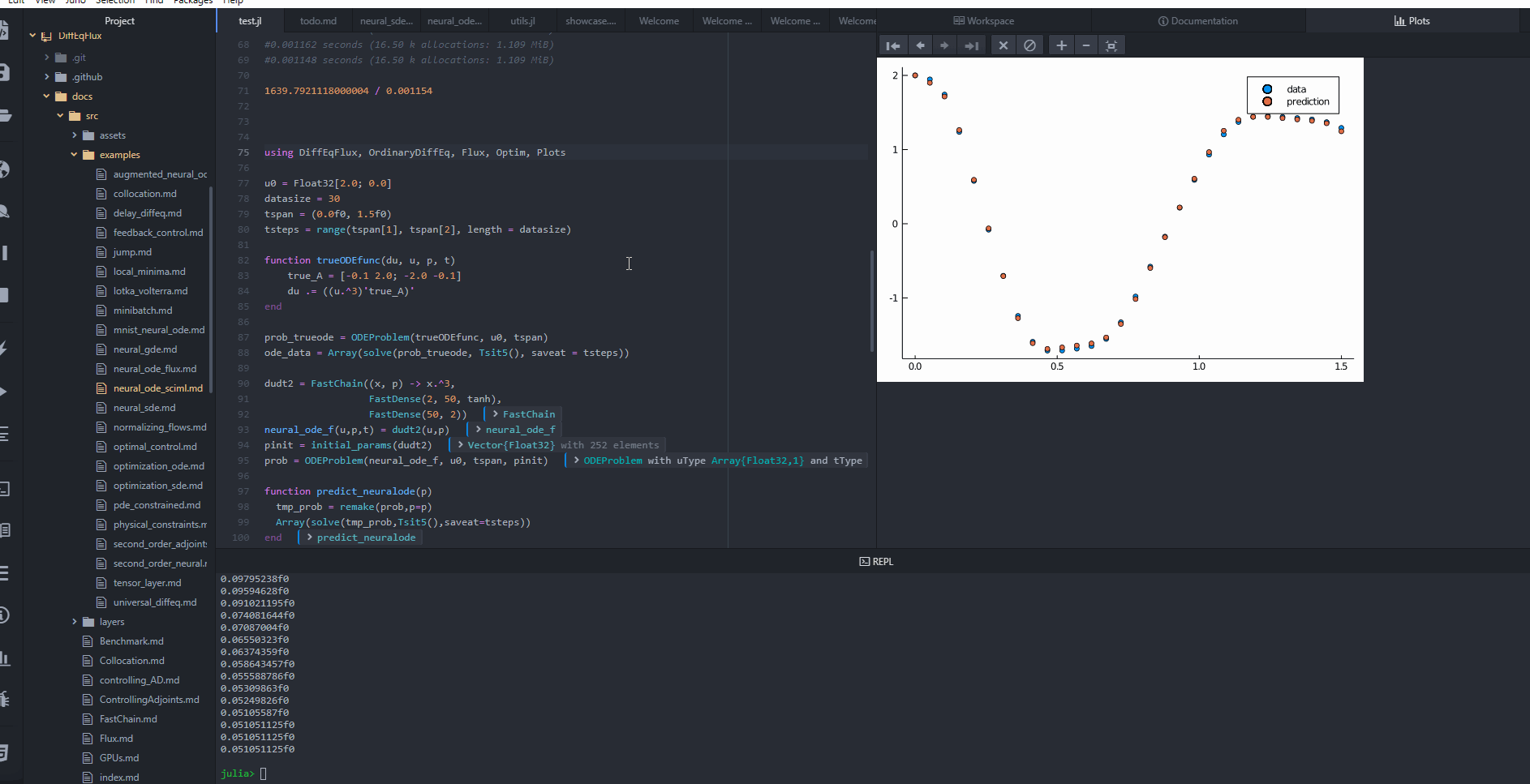# Neural Ordinary Differential Equations with GalacticOptim.jl

DiffEqFlux.jl defines sciml_train which is a high level utility that automates a lot of the choices, using heuristics to determine a potentially efficient method. However, in some cases you may want more control over the optimization process. The underlying optimization package behind sciml_train is GalacticOptim.jl. In this tutorial we will show how to more deeply interact with the optimization library to tweak its processes.

We can use a neural ODE as our example. A neural ODE is an ODE where a neural network defines its derivative function. Thus for example, with the multilayer perceptron neural network FastChain(FastDense(2, 50, tanh), FastDense(50, 2)), we obtain the following results.

## Copy-Pasteable Code

Before getting to the explanation, here's some code to start with. We will follow a full explanation of the definition and training process:

using DiffEqFlux, DifferentialEquations, Plots, GalacticOptim

u0 = Float32[2.0; 0.0]
datasize = 30
tspan = (0.0f0, 1.5f0)
tsteps = range(tspan, tspan, length = datasize)

function trueODEfunc(du, u, p, t)
true_A = [-0.1 2.0; -2.0 -0.1]
du .= ((u.^3)'true_A)'
end

prob_trueode = ODEProblem(trueODEfunc, u0, tspan)
ode_data = Array(solve(prob_trueode, Tsit5(), saveat = tsteps))

dudt2 = FastChain((x, p) -> x.^3,
FastDense(2, 50, tanh),
FastDense(50, 2))
prob_neuralode = NeuralODE(dudt2, tspan, Tsit5(), saveat = tsteps)

function predict_neuralode(p)
Array(prob_neuralode(u0, p))
end

function loss_neuralode(p)
pred = predict_neuralode(p)
loss = sum(abs2, ode_data .- pred)
return loss, pred
end

callback = function (p, l, pred; doplot = true)
display(l)
# plot current prediction against data
plt = scatter(tsteps, ode_data[1,:], label = "data")
scatter!(plt, tsteps, pred[1,:], label = "prediction")
if doplot
display(plot(plt))
end
return false
end

# use GalacticOptim.jl to solve the problem

optf = GalacticOptim.OptimizationFunction((x, p) -> loss_neuralode(x), adtype)
optfunc = GalacticOptim.instantiate_function(optf, prob_neuralode.p, adtype, nothing)
optprob = GalacticOptim.OptimizationProblem(optfunc, prob_neuralode.p)

result_neuralode = GalacticOptim.solve(optprob,
cb = callback,
maxiters = 300)

optprob2 = remake(optprob,u0 = result_neuralode.u)

result_neuralode2 = GalacticOptim.solve(optprob2,
LBFGS(),
cb = callback,
allow_f_increases = false)## Explanation

Let's get a time series array from the Lotka-Volterra equation as data:

using DiffEqFlux, DifferentialEquations, Plots

u0 = Float32[2.0; 0.0]
datasize = 30
tspan = (0.0f0, 1.5f0)
tsteps = range(tspan, tspan, length = datasize)

function trueODEfunc(du, u, p, t)
true_A = [-0.1 2.0; -2.0 -0.1]
du .= ((u.^3)'true_A)'
end

prob_trueode = ODEProblem(trueODEfunc, u0, tspan)
ode_data = Array(solve(prob_trueode, Tsit5(), saveat = tsteps))

Now let's define a neural network with a NeuralODE layer. First we define the layer. Here we're going to use FastChain, which is a faster neural network structure for NeuralODEs:

dudt2 = FastChain((x, p) -> x.^3,
FastDense(2, 50, tanh),
FastDense(50, 2))
prob_neuralode = NeuralODE(dudt2, tspan, Tsit5(), saveat = tsteps)

Note that we can directly use Chains from Flux.jl as well, for example:

dudt2 = Chain(x -> x.^3,
Dense(2, 50, tanh),
Dense(50, 2))

In our model we used the x -> x.^3 assumption in the model. By incorporating structure into our equations, we can reduce the required size and training time for the neural network, but a good guess needs to be known!

From here we build a loss function around it. The NeuralODE has an optional second argument for new parameters which we will use to iteratively change the neural network in our training loop. We will use the L2 loss of the network's output against the time series data:

function predict_neuralode(p)
Array(prob_neuralode(u0, p))
end

function loss_neuralode(p)
pred = predict_neuralode(p)
loss = sum(abs2, ode_data .- pred)
return loss, pred
end

We define a callback function.

# Callback function to observe training
callback = function (p, l, pred; doplot = false)
display(l)
# plot current prediction against data
plt = scatter(tsteps, ode_data[1,:], label = "data")
scatter!(plt, tsteps, pred[1,:], label = "prediction")
if doplot
display(plot(plt))
end
return false
end

We then train the neural network to learn the ODE.

Here we showcase starting the optimization with ADAM to more quickly find a minimum, and then honing in on the minimum by using LBFGS. By using the two together, we are able to fit the neural ODE in 9 seconds! (Note, the timing commented out the plotting). You can easily incorporate the procedure below to set up custom optimization problems. For more information on the usage of GalacticOptim.jl, please consult this documentation.

The x and p variables in the optimization function are different than x and p above. The optimization function runs over the space of parameters of the original problem, so x_optimization == p_original.

# Train using the ADAM optimizer

optf = GalacticOptim.OptimizationFunction((x_optimization, p_optimization) -> loss_neuralode(x_optimization), adtype)
optfunc = GalacticOptim.instantiate_function(optf, prob_neuralode.p, adtype, nothing)
optprob = GalacticOptim.OptimizationProblem(optfunc, prob_neuralode.p)

result_neuralode = GalacticOptim.solve(optprob,
cb = callback,
maxiters = 300)
# output
* Status: success

* Candidate solution
u: [4.38e-01, -6.02e-01, 4.98e-01,  ...]
Minimum:   8.691715e-02

* Found with
Initial Point: [-3.02e-02, -5.40e-02, 2.78e-01,  ...]

We then complete the training using a different optimizer starting from where ADAM stopped. We do allow_f_increases=false to make the optimization automatically halt when near the minimum.

# Retrain using the LBFGS optimizer
optprob2 = remake(optprob,u0 = result_neuralode.u)

result_neuralode2 = GalacticOptim.solve(optprob2,
LBFGS(),
cb = callback,
allow_f_increases = false)
# output
* Status: success

* Candidate solution
u: [4.23e-01, -6.24e-01, 4.41e-01,  ...]
Minimum:   1.429496e-02

* Found with
Algorithm:     L-BFGS
Initial Point: [4.38e-01, -6.02e-01, 4.98e-01,  ...]

* Convergence measures
|x - x'|               = 1.46e-11 ≰ 0.0e+00
|x - x'|/|x'|          = 1.26e-11 ≰ 0.0e+00
|f(x) - f(x')|         = 0.00e+00 ≤ 0.0e+00
|f(x) - f(x')|/|f(x')| = 0.00e+00 ≤ 0.0e+00
|g(x)|                 = 4.28e-02 ≰ 1.0e-08

* Work counters
Seconds run:   4  (vs limit Inf)
Iterations:    35
f(x) calls:    336
∇f(x) calls:   336